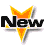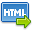Journal :   International Journal of Technology

Volume No. :   6

Issue No. :  2

Year :  2016

Pages :   133-137

ISSN Print :  2231-3907

ISSN Online :  2231-3915Registration

## Math in Chemistry and Economics

 Address: Dr. Anil Kumar Sharma, Dr. Minakshi 1Deptt. of Chemistry Govt. College Bhoranj (Tarkwari) Distt. Hamirpur (H.P.) 2Deptt. of Economics Govt. Degree College Barotiwala Distt. Solan (H.P.) *Corresponding Author
DOI No: 10.5958/2231-3915.2016.00021.3

ABSTRACT:
Now a days math is having its own importance in different fields of science, Engineering, Medical Science, Economics, Commerce etc. All the fields have been developed by making use of math. In this paper some uses of math in the field of chemistry and Economics are highlighted. In the field of chemistry for different experimental results which are calculated by making use of math. For getting the same value of experimental results by making use of mathematically calculated theories are done. It is found that experimental results and the result obtained by theories resemble with each other. So without doing experiments, only by mathematical theories the results can be calculated e.g. experimental results of excess volume can also be calculated from Flory’s statistical theory and Prigogine- Flory’s Patterson theory, Hydrodynamic and osmotic permeability. Electro- osmotic permeability Thermodynamic studies from electromotive force measurements, Electro negativity calculation etc. are explained in this paper. An idea about use of math in Economics is proposed by explaining some theories e.g. Marginal utility, law of equimarginal utility marginal rate of substitution, marginal lost, Harodommars model etc.
KEYWORDS:
Math in Chemistry and Economics
Cite:
Anil Kumar Sharma, Minaksh. Math in Chemistry and Economics. Int. J. Tech. 2016; 6(2): 133-137[View HTML][View PDF]

Visitor's No. :   125044